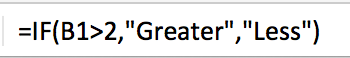# The Excel Skinny

A blog about Excel and its users

## Using Logical Formulas in Microsoft Excel

September 28, 2016

## How do you compare data in Microsoft Excel? Logical Formulas!

Excel uses logical operations to ease the pain in comparing data. These formulas will return what we tell them to, based on specific criteria.

We will teach you how to run less than, greater than, and equal to formulas below.
For the first formula, we will focus on the “Greater Than” formula. This is used when you want to isolate cells that contain values greater than the parameters in your formula.
For the example below, I have a series of numbers between 1 and 10. All I want to know is if the number is greater than, or less than 2.As shown above, my formula is: =IF(B1>2,"Greater","Less") for the first cell. This is telling Excel, if the data in B1 is greater than 2, input the word “Greater”. If it is less than 2, input “Less”.  I then pulled this formula down by the square in the lower right hand side of the D1 cell to fit my list.

This is a very simple way to use the =IF logical formulas, but they can become extremely complex if needed.

As always, please let the Excel Skin team know if you have any questions!